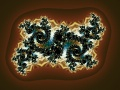# Writing formulasIn Ultra Fractal, every part of the fractal calculation process is controlled by formulas. There are three types of formulas: fractal formulas, coloring algorithms, and transformations. A formula can be seen as a small, specialized computer program that is compiled and executed by Ultra Fractal.

By writing your own formulas, you can completely customize how a fractal is calculated. It is recommended to first learn how to use Ultra Fractal and get comfortable with it before you start writing formulas.

This chapter explains how to write your own formulas. It is divided into five sections:

• The Language basics section introduces the syntax and elements of the formula language. You should study this first.
• The Functions and classes section describes how to declare and use your own functions and classes, including inheritance and plug-ins.
• The Formulas section shows how to use the formula language in practice to write fractal formulas, coloring algorithms, and transformations.
• The Reference section describes all operators, built-in functions, predefined symbols, etc. that are available.
• The Tips section contains additional information on debugging and publishing your formulas.

Use the Contents tab to navigate to topics in these sections.